Reconstruction of the 2015 Blackhorse Lane formation 1.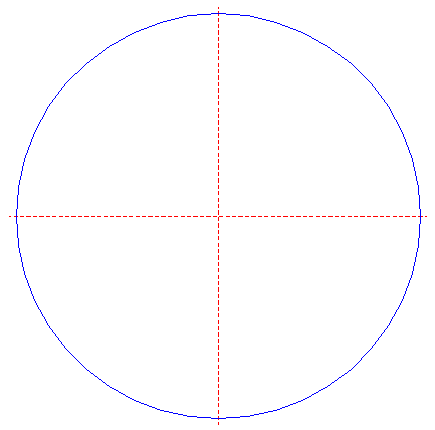Draw a circle. Draw and extend the horizontal and vertical centerlines. 2.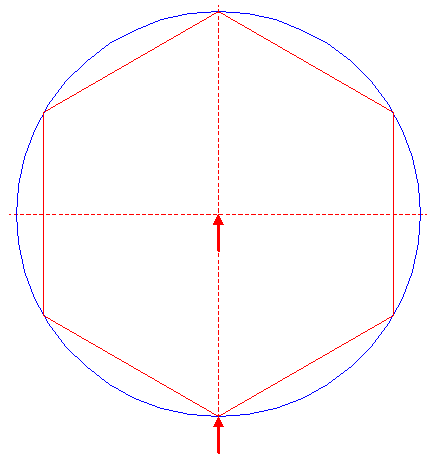Construct the inscribed hexagon (regular 6-sided polygon) of circle 1, pointing down. 3.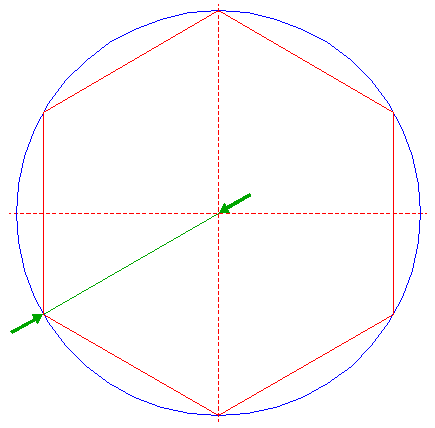Draw the ray of hexagon 2, from the center of circle 1 to its lower lefthand angular point. 4.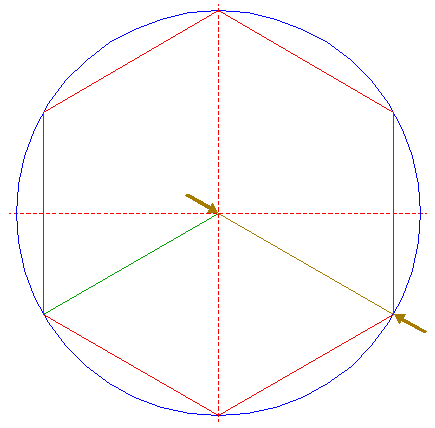Draw the ray of hexagon 2, from the center of circle 1 to its lower righthand angular point. 5.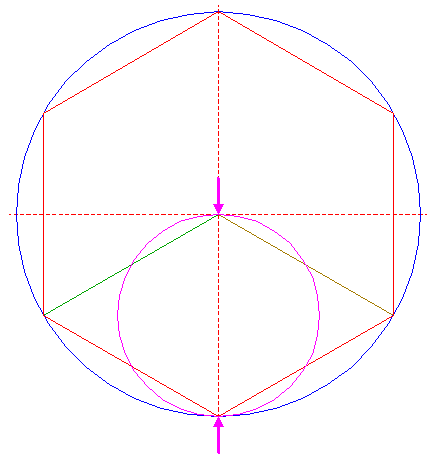Construct a "two-points" circle (defined by the two end-points of a centerline) between the center of circle 1 and its lower intersection with the vertical centerline. 6.Construct two "two-points" circles, between the center of circle 5 and each of its intersections with the vertical centerline. 7.Construct three circles concentric to circle 1, passing through the centers of circles 5 and 6. 8.Copy circles 7 to the lower intersection of circle 1 and the vertical centerline. 9.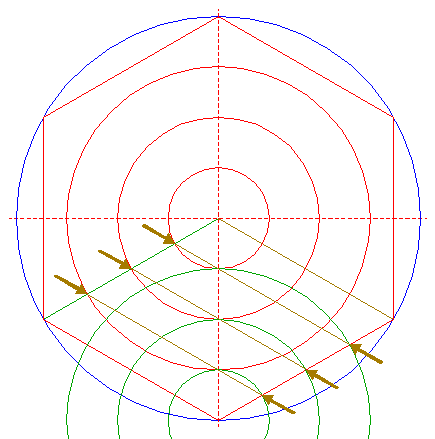Draw three parallel lines, from the intersections of circles 7 and line 3 to the intersections of circles 8 and the lower righthand side of hexagon 2. 10.Draw three parallel lines, from the intersections of circles 7 and line 4 to the intersections of circles 8 and the lower lefthand side of hexagon 2. 11.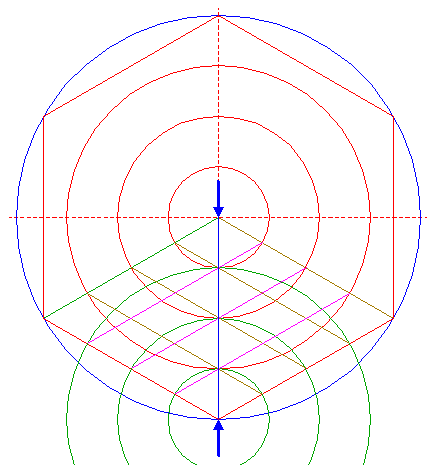Draw a line from the center of circle 1 to its lower intersection with the vertical centerline. 12.Draw three vertical lines from the intersections of lines 9 and line 3 to the intersections of lines 10 and the lower lefthand side of hexagon 2. 13.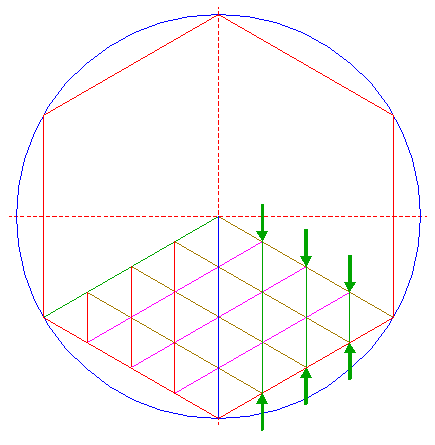Draw three vertical lines from the intersections of lines 10 and line 4 to the intersections of lines 9 and the lower righthand side of hexagon 2. 14.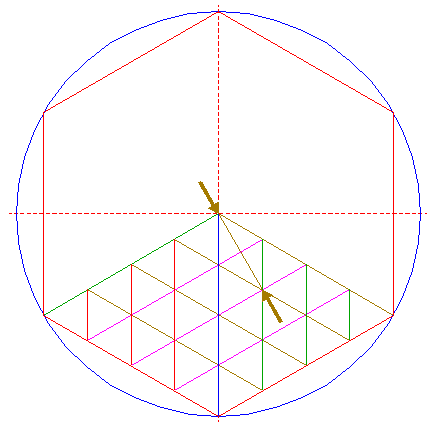Draw a line from the center of circle 1 to the intersection of the upper line 9 and the middle line 10. 15.Construct a circle centered at the intersection of the upper line 10 and line 14, tangent to the innermost circle 7 at the lower righthand side. 16.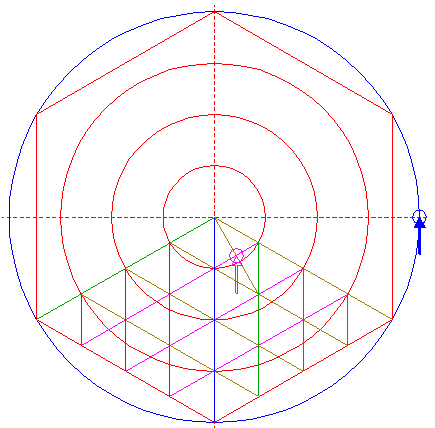Copy circle 15 to the righthand intersection of circle 1 and the horizontal centerline. 17.Construct a circle concentric to circle 1, tangent to circle 16 at the righthand side. 18.Construct a "two-points" circle between the center of circle 15 and its lower intersection with line 14. 19.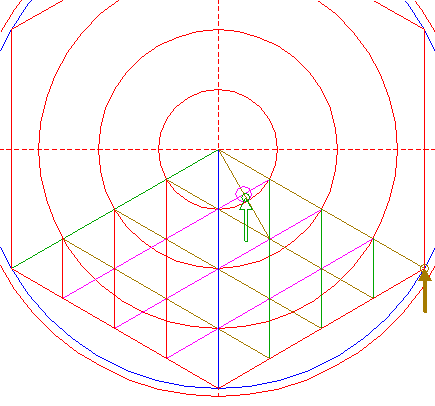Copy circle 18 to the lower righthand angular point of hexagon 2. 20.Copy circle 18 to the center of circle 1. 21.Draw a line parallel to line 4, tangent to circles 19 and 20 at the lower lefthand sides. 22.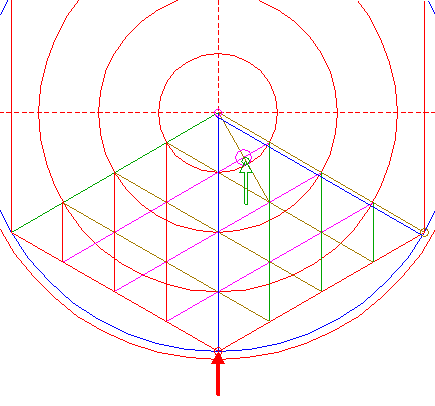Copy circle 18 to the lower angular point of hexagon 2. 23.Draw a line parallel to the lower righthand side of hexagon 2, tangent to circles 19 and 22 at the upper lefthand sides. 24.Circles 1 and 17, hexagon 2, and lines 3, 4, 9, 10, 11, 12, 13, 21, and 23, are used for the final reconstruction. 25.Remove all parts not visible within the formation itself. 26.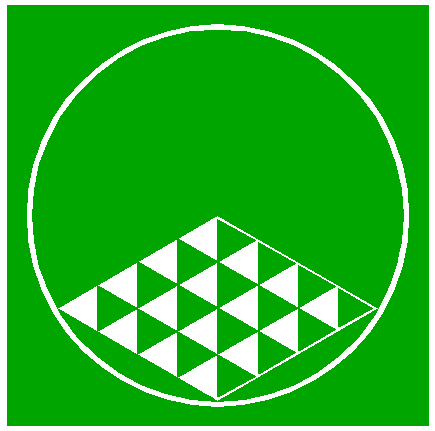Colour all areas corresponding to standing... 27....or to flattened crop, and finish the reconstruction of the 2015 Blackhorse Lane formation. 28.The final result, matched with the aerial image.